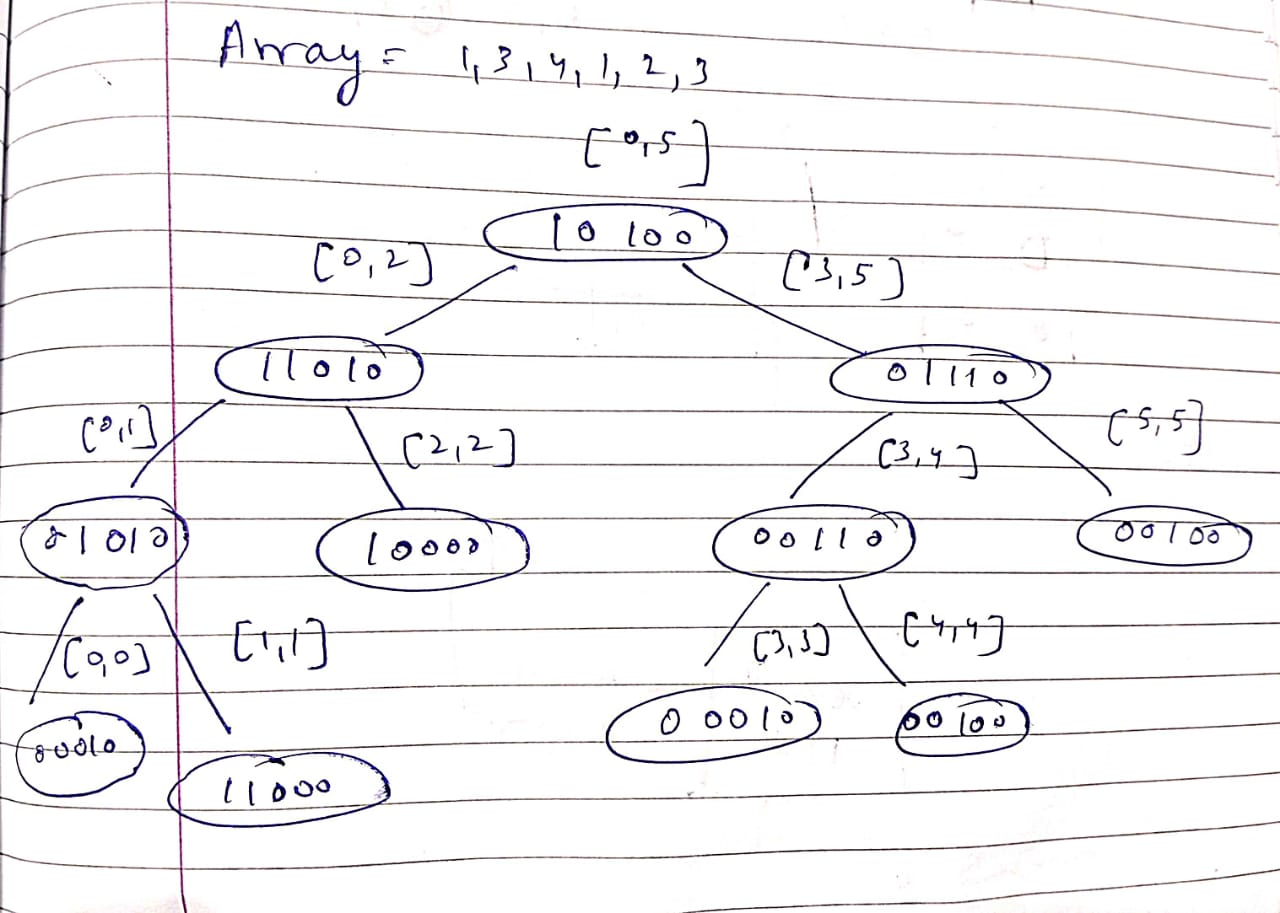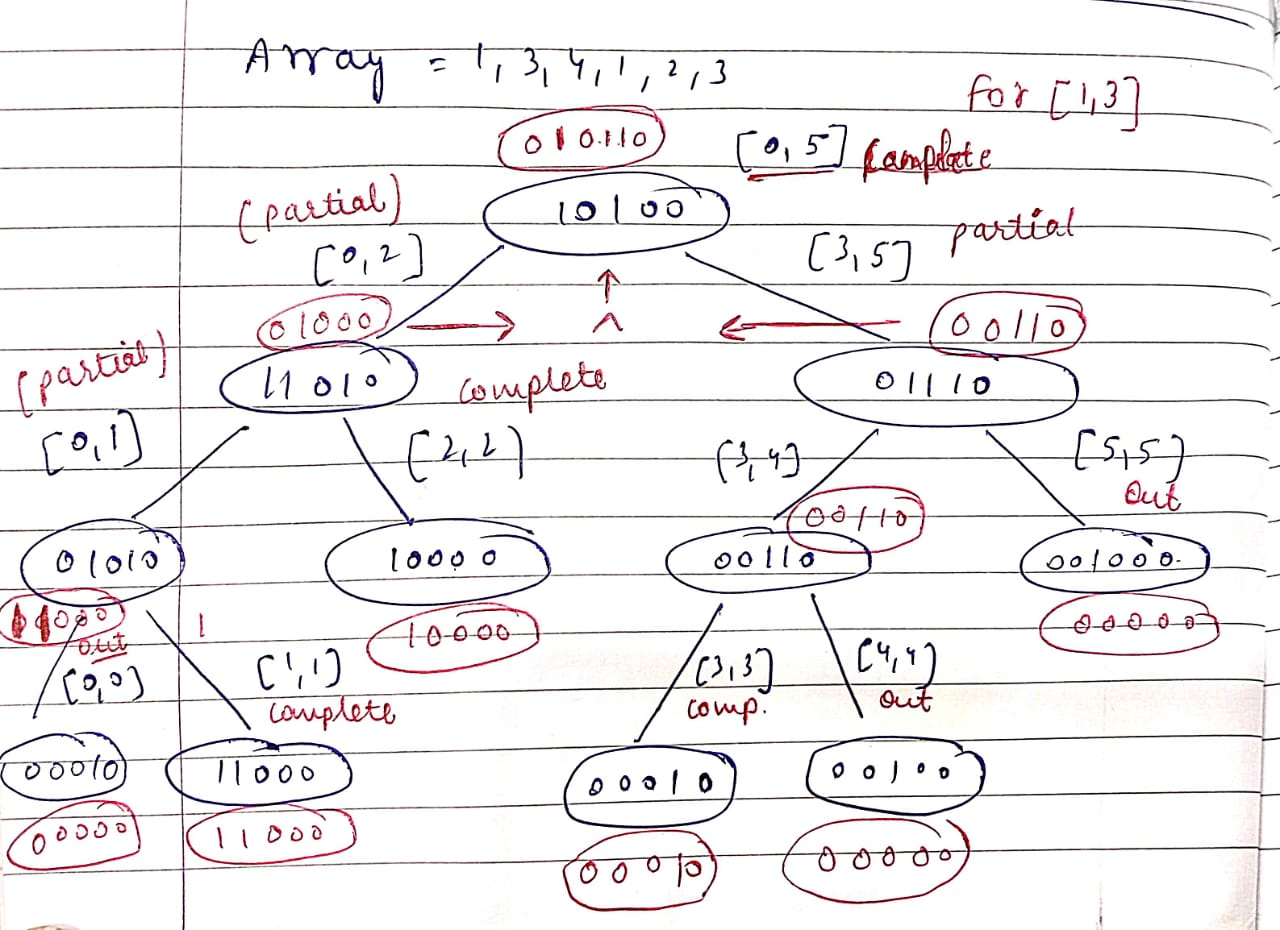# LVFODD - Editorial

Author: Abhishek Ghosh
Tester: Abhishek Ghosh
Editorialist: Meenal Beniwal, Priyam Agarwal

Medium

# PREREQUISITES

Segment Tree, Bit Manipulation

# PROBLEM:

There is an array A consisting of N elements and we are given Q queries to perform which would be of the following 3 types-

• ? L R - Output the number of unique elements that Chefina doesn’t like in the complete array. The condition for liking the element is that the frequency of the element is odd in the given index range [L,R].

• * i X - Replace the i^{th} index element with X.

• ! i Y - Replace the i^{th} index element with the GCD of A_i and Y.

# EXPLANATION

We have two approaches for the problem, the first one being a naïve one as it will only work for subtask 1 and will give TLE verdict for subtask 2. So, we require a better approach to the problem.
We will require an understanding of Segment Trees and Bitsets to solve this problem.

### Naïve Approach:

Using frequency array, with a worst case time complexity of \mathcal O(Q*N)

Naïve Approach

We can create a frequency array to store the frequency of the elements and also keep track of the number of unique elements in another variable.

• For 1st Query:

We can iterate over the given range [L,R] for each query and create a frequency array for each query, and further count the number of elements that appear an odd number of times and subtract it from the unique elements count.

• For 2nd and 3rd Query:

We can simply replace the values. If all the queries are of type 1, then it will consume a lot of time.

Hence, we need to come up with a better solution.

### Better Approach:

We will be creating a segment tree to store the number of elements that appear an odd number of times in a particular segment.

Segment tree overview

At the first step, a segment tree is formed. We follow 1-base indexing in the segment tree.
If the root node is at i^{th} index then-

• Left Child will be at 2*i position and
• Right Child will be at 2*i +1 position.

A recursive function will be used to build the segment tree in \mathcal O(N) time.

In the segment tree, each node represents the answer for the corresponding range using bitset. Since the range of A_i is [0,10^3), we can use a bitset of size 1000 bits.

The leaf node represents an element of the array (the X^{th} bit will be set at leaf node if the element in the array is X). The parent node is obtained by taking XOR of its children nodes.

But why XOR?

If an element appears an odd number of times in the left range as well as the right range, then in the complete range it will appear an even number of times represented by resetting the bit to 0.
Following this logic, it is clear that if the frequency of an element has same parity in left and right ranges, then its corresponding bit will be 0, otherwise it will be 1. This is nothing but the XOR of both sides. Bitsets in C++ provide us with this bitwise functionality and we can simply take the XOR of the left and right child to compute the answer for parent node, (or the current range).

Time taken will be \mathcal O(\frac{D}{32}), where D is the size of the bitset.

#### For 1st Query:

The node would be a D bit binary number (or a bitset) for the range of that node where,

• 0 at i^{th} index would represent that the element “i” is either not present in that range, or is present even number of times, whereas
• 1 at i^{th} index represents that the element is present an odd number of times.

Bitset follows 0-base indexing from right to left.
For example, in a 4-bit binary number 0010, this represents even occurrences of 3,2,0 and odd occurrences of 1.

The range of the tree: It is the part from which the query is executed.

Example

a[]=[1,3,4,1,2,3] size from [0,5] (this is the range) i.e root of tree;Left subtree will store the answer for the range [0,2] and the right subtree for [3,5] (these are the ranges).
In this way, we continue to form the segment tree. The leaf node for the range [0,0] i.e the first element is 00010, and [1,1] represents 01000 (wrongly mentioned in the figure as 11000). The parent element i.e [0,1] will be the XOR of its children i.e 01010.To solve the query,
Frequency bitset is found for the given range. For instance, the range is [1,3], as shown in the above example. Then we have 3 possible cases.

• Case 1: (No overlap)
When it lies outside the range of the parent node:
In this case, return the value [00000...000] via bitset.
• Case 2: (Complete overlap)
When it lies completely inside the range of the parent node:
Return the bitset present here, without exploring further children.
• Case 3: (Partial overlap)
In this case take the XOR of the value of both nodes.
In the example above, we have [1,3]
Starting from the root node [0,5], the left subtree is [0,2] and right subtree is [3,5].
Hence [1,3] lies partially in both. Therefore, continue till leaf node and take respective XOR till we get the answer for both ranges [0,2] and [3,5]. Finally the output will be the XOR of the two children.

#### For 2nd and 3rd Query:

To update the tree,
If we have to replace the value of any element in the array then it would mean that a leaf node of that element has to be updated and hence there has to be changes for all those nodes that appear from that leaf node all the way up to the root node.
So after replacing the value of the leaf node, we go on to update its parent node by taking the XOR of the child nodes and so on till the root node (similar to the first query).
This can be done in a bottom-up fashion for both the queries as they require only updation and are virtually the same. Additional \mathcal O(log(maxA_i)) work has to be done for calculating GCD.

### Time Complexity

\mathcal O(N * log(A_i) + Q * (log(A_i) + log(N))*(\frac{D}{32}) )
Where, D=1000 as given in the problem (upper bound for A_i).

# SOLUTIONS

Setter's Solution
#include <bits/stdc++.h>
using namespace std;

#define SIZE 1000
bitset<SIZE> segtree;

void build_tree(vector<int>& arr, int st, int en, int curr) {
if(st == en) {
segtree[curr].reset();
segtree[curr].set(arr[st]);
return;
}
int mid = st + (en-st) / 2;
int l_child = 2*curr;
int r_child = 2*curr + 1;
build_tree(arr, st, mid, l_child);
build_tree(arr, mid+1, en, r_child);
segtree[curr] = (segtree[l_child] ^ segtree[r_child]);
}

void update_tree(vector<int>& arr, int st, int en, int curr, int idx, int value) {
if(idx < st or idx > en) {
return;
}
if(st == en) {
segtree[curr].reset();
segtree[curr].set(value);
arr[idx] = value;
return;
}
int mid = st + (en-st) / 2;
int l_child = 2*curr;
int r_child = 2*curr + 1;
update_tree(arr, st, mid, l_child, idx, value);
update_tree(arr, mid+1, en, r_child, idx, value);
segtree[curr] = (segtree[l_child] ^ segtree[r_child]);
}

bitset<SIZE> query(int st, int en, int curr, int l, int r) {
if(st > r || en < l) {
bitset<SIZE> b;
return b;
}
if(st >= l and en <= r) {
return segtree[curr];
}
int mid = st + (en-st) / 2;
int l_child = 2*curr;
int r_child = 2*curr + 1;
auto x = query(st, mid, l_child, l, r);
auto y = query(mid+1, en, r_child, l, r);
return (x ^ y);
}

int main(){
#ifndef ONLINE_JUDGE
freopen("input.txt", "r", stdin);
freopen("output.txt", "w", stdout);
#endif
ios_base::sync_with_stdio(false);
cin.tie(NULL);
cout.tie(NULL);

unordered_map<int, int> freq;
bitset<SIZE> b;
int n; cin >> n;
vector<int> arr(n);
for(int &x : arr) {
cin >> x;
freq[x]++;
b.set(x);
}

build_tree(arr, 0, n-1, 1);

int q; cin >> q;
while(q--) {
char type; cin >> type;
if(type == '?') {
int l, r;
cin >> l >> r;
auto bits = query(0, n-1, 1, l-1, r-1);
int ans = (bits^b).count();
cout << ans << "\n";
} else {
int idx, val;
cin >> idx >> val;
idx--;
if(type == '!') {
val = __gcd(arr[idx], val);
}
freq[arr[idx]]--;
if(freq[arr[idx]] == 0) {
b.reset(arr[idx]);
}
if(freq[val] == 0) {
b.set(val);
}
freq[val]++;
update_tree(arr, 0, n-1, 1, idx, val);
}
}
return 0;
}